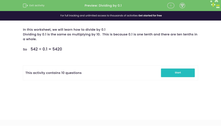# Divide by 0.1

In this worksheet, students will practise dividing numbers by 0.1, understanding that this is the same as multiplying by 10.Key stage:  KS 3

Curriculum topic:   Number

Curriculum subtopic:   Use Four Operations for All Numbers

Popular topics:   Multiplication worksheets, Division worksheets

Difficulty level:#### Worksheet Overview

In this activity, we will learn how to divide by 0.1

Dividing by 0.1 is the same as multiplying by 10.  This is because 0.1 is one tenth and there are ten tenths in a whole.

so, 542 ÷ 0.1 = 5,420 and also 542 x 10 = 5,420

Simple, isn't it?!

2 ÷ 0.1

This is the same as 2 x 10 = 20

and also 0.35 ÷ 0.1 is the same as 0.35 x 10 = 3.5

Shall we have a go at some questions now?### What is EdPlace?

We're your National Curriculum aligned online education content provider helping each child succeed in English, maths and science from year 1 to GCSE. With an EdPlace account you’ll be able to track and measure progress, helping each child achieve their best. We build confidence and attainment by personalising each child’s learning at a level that suits them.

Get started